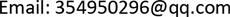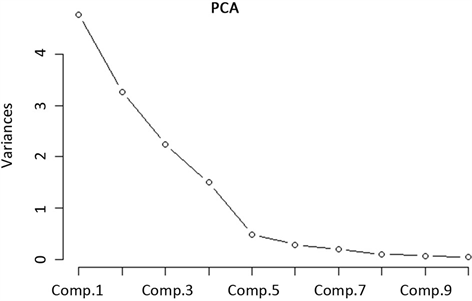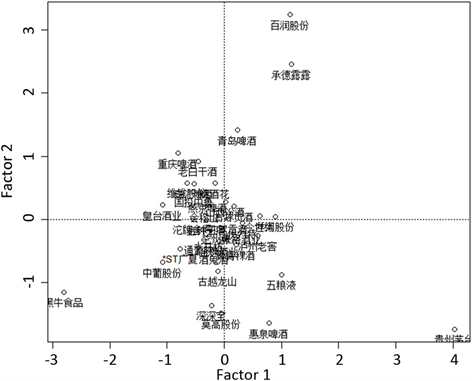﻿ 酒类上市公司经营绩效实证分析及R实现 Empirical Analysis and R Realization of Business Performance of Alcohol Listed Companies

Statistics and Application
Vol.07 No.04(2018), Article ID:26498,12 pages
10.12677/SA.2018.74049

Empirical Analysis and R Realization of Business Performance of Alcohol Listed Companies

Miao Wang

College of Economics, Jinan University, Guangzhou GuangdongReceived: Jul. 27th, 2018; accepted: Aug. 12th, 2018; published: Aug. 20th, 2018ABSTRACT

The operating performance of listed companies is of great significance for attracting investment and adjusting corporate strategy. China’s liquor listed companies are in the stage of diversification and expansion, and it is indispensable to quantitatively analyze the indicators reflecting the performance level of alcoholic enterprises. This paper conducts empirical research on 37 liquor listed companies, uses R language as the realization tool, selects the main financial indicators to construct the performance evaluation model, uses factor analysis method to sort and score the enterprise performance, and uses cluster analysis method to different performance levels. The liquor companies are classified hierarchically. According to the empirical analysis of performance, the ability of investor risk identification is improved and corresponding countermeasures for enterprise development are formulated.

Keywords:Alcohol Listed Company, Performance Evaluation, Factor Analysis, Cluster Analysis1. 引言

2. 模型简述

2.1. 因子分析模型

1) 将原始数据标准化

2) 建立变量的相关系数矩阵r

${r}_{ij}=\frac{\sum _{a=1}^{n}\left({x}_{ai}-{\overline{x}}_{i}\right)\left({x}_{aj}-{\overline{x}}_{j}\right)}{\sqrt{\sum _{a=1}^{n}{\left({x}_{ai}-{\overline{x}}_{i}\right)}^{2}}\cdot \sqrt{\sum _{a=1}^{n}{\left({x}_{aj}-{\overline{x}}_{j}\right)}^{2}}}=\frac{1}{n}\sum _{a=1}^{n}{x}_{ai}{x}_{aj}$ (1)

3) 求r的特征根和相应的单位特征向量

$U=\left({u}_{1},{u}_{2},\cdots ,{u}_{p}\right)=\left(\begin{array}{cccc}{u}_{11}& {u}_{12}& \cdots & {u}_{1p}\\ {u}_{21}& {u}_{22}& \cdots & {u}_{2p}\\ ⋮& ⋮& & ⋮\\ {u}_{p1}& {u}_{p1}& \cdots & {u}_{pp}\end{array}\right)$ (2)

4) 确定因子个数，建立因子载荷矩阵

$A=\left(\begin{array}{cccc}{a}_{11}& {a}_{12}& \cdots & {a}_{1m}\\ {a}_{21}& {a}_{22}& \cdots & {a}_{2m}\\ ⋮& ⋮& & ⋮\\ {a}_{p1}& {a}_{p1}& \cdots & {a}_{pm}\end{array}\right)=\left(\begin{array}{cccc}{\tau }_{11}\sqrt{{\lambda }_{1}}& {\tau }_{12}\sqrt{{\lambda }_{2}}& \cdots & {\tau }_{1m}\sqrt{{\lambda }_{m}}\\ {\tau }_{21}\sqrt{{\lambda }_{1}}& {\tau }_{22}\sqrt{{\lambda }_{2}}& \cdots & {\tau }_{2m}\sqrt{{\lambda }_{m}}\\ ⋮& ⋮& & ⋮\\ {\tau }_{p1}\sqrt{{\lambda }_{1}}& {\tau }_{p2}\sqrt{{\lambda }_{2}}& \cdots & {\tau }_{pm}\sqrt{{\lambda }_{m}}\end{array}\right)$ (3)

5) 对因子载荷矩阵进行因子旋转

6) 估计因子得分

7) 综合得分

2.2. 聚类分析方法

1) 数据预处理。在聚类分析中，要求被选中的进行分析的全部指标变量的量纲应该一致，在聚类分析前应该视指标的量纲情况来决定是否要先对数据进行处理。常用的整理原始数据的方法有：数据中心变换法、数据标准化变换法、极差正规化变换法、对数变换法。本文主要采用数据标准化变换法。

2) 相似性度量。聚类分析首先应该对各变量进行相似性度量，有距离和相似系数两个常用方法，在相似系数法中经常用的有相关系数和夹角余弦等方法。变量的测量方法因测量尺度不同而不同，通常情况下用距离对样品进行聚类，而用相似系数对变量进行聚类。

3) 选择聚类方法。确定样品相似性或不相似性的统计量之后，就要对样品或变量进行分类。样品聚类和变量聚类的方法很多，通常使用的有系统聚类法，模糊聚类法，K-均值法等。系统聚类分析的类型依距离定义的类型而定，在实际中Ward离差平方和法和组间平均连接法应用较多。

3. 实证分析

3.1. 数据获取及指标体系构建

3.2. 数据的预处理

${z}_{ij}=\frac{{x}_{ij}-{\overline{x}}_{i}}{{s}_{j}}$ (4)

3.3. 适度性检验

3.4. 因子分析

3.4.1. 提取公因子Table 1. Comprehensive evaluation index system for liquor listed companies

3.4.2. 建立因子载荷矩阵

3.4.3. 因子命名

1) 公因子F1的因子载荷主要分布在总资产报酬率、净利润率、固定资产周转率和速动比率上，与之前根据巨潮资讯所给定的公司综合能力指标不同，固定资产周转率载荷较为突出，这反映出了盈利能力

2) 公因子F2在总资产周转率、资产负债比率和每股净资产上的载荷较高，与之前所给的指标分类相比较，因子分类以后公司的扩张能力、偿债能力和经营能力表现出一定的关联性，这两种能力可以归结为公司的发展能力因子。

3) 公因子F3在存货周转率、流动比率和应收账款周转率上具有较大加大的载荷，经营能力和偿债能力有一定的关联，但其中存货周转率载荷远高于其它两个指标，所以可以认为该因子为公司的经营能力因子。

4) 因子F4在每股收益、净资产收益率和扣除后每股收益上的载荷较大，分别反映上市公司给予其股东的回报，在这个因子上得分越高，则公司能够给予股东的回报一般而言也越高。Table 3. List of eigenvalues and their contribution ratesFigure 1. Screen plotTable 4. Load matrix of factors before and after rotationTable 5. Main factor naming and distribution map

3.4.4. 计算因子得分和排名

${F}_{j}={a}_{j1}{x}_{1}+{a}_{j2}{x}_{2}+\cdots +{a}_{jp}{x}_{p},\text{\hspace{0.17em}}\text{\hspace{0.17em}}j=1,2,\cdots ,m$ (5)Table 6. Scores and rankings of various factors in 37 liquor listed companiesFigure 2. Graph of factor scoresTable 7. Comprehensive scores and rankings of 37 liquor listed companies

$F=\frac{{\lambda }_{1}{F}_{1}+{\lambda }_{2}{F}_{2}+\cdots +{\lambda }_{m}{F}_{m}}{{\lambda }_{1}+{\lambda }_{2}+\cdots +{\lambda }_{m}}=\sum _{i=1}^{m}{w}_{i}{F}_{i}$ (6)

$F=\frac{{F}_{1}×26.26+{F}_{2}×23.65+{F}_{3}×21.36+{F}_{4}×19.37}{90.65}$ (7)

3.5. 聚类分析

4. 结论

1) 多方面协调发展。根据实证分析，业绩突出的公司多项因子均排名靠前，证明酒类公司单一发展，应重视多方面协调发展以及实现各种资源的合理有效配置，进而提升公司综合竞争能力。

2) 提升盈利能力，保证收益质量和加强资产管理水平。资金运行良好是企业长期稳定发展和提升企业核心竞争力的重要保障，稳定的盈利能力是评价企业竞争力的重要评价指标。

3) 酒类行业集群展。集群经济有利于酒类公司降低成本，加强整个产业的专业化，提高创新能力，打造品牌效应。同时，改进产品的质量，以市场需求为导向开发多样化酒类品牌，拓展国外市场。上市公司应该充分利用有关政策的支持和行业渠道开放的机会，打破传统的代理销售渠道，积极发展电商模式。

Empirical Analysis and R Realization of Business Performance of Alcohol Listed Companies[J]. 统计学与应用, 2018, 07(04): 426-437. https://doi.org/10.12677/SA.2018.74049

1. 1. 朱华琳. 我国寿险公司偿付能力监管指标的因子分析[J]. 统计与决策, 2015(2): 186-188.

2. 2. 徐占东. 基于聚类分析的商业银行效率评价模型研究[J]. 统计与决策, 2015(4): 158-163.

3. 3. 张若钦. 基于Malmquist指数的白酒类上市公司效率分析[J]. 时代经贸, 2008, 6(8): 21-22.

4. 4. 胡燕京, 冯琦. 基于DEA的我国上市旅游企业经营绩效评价[J]. 华东经济管理, 2006, 20(9): 62-65.

5. 5. 徐蔼婷. 德尔菲法的应用及其难点[J]. 中国统计, 2006(9): 57-59.

6. 6. 王伟. 我国P2P网络借贷平台风险评价——基于熵权法和CRITIC法[J]. 金融理论与实践, 2016(12).

7. 7. 张先治, 李琦. 基于EVA的业绩评价对央企过度投资行为影响的实证分析[J]. 当代财经, 2012(5): 119-128.

8. 8. 孙丽华. 基于灰色关联模型的中国林业上市公司绩效评价研究[J]. 林业经济问题, 2013, 33(4): 283-288.